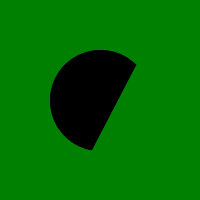# Wand arc() function in Python

arc() is a function present in wand.drawing module. arc() function draws an arc in the image. You’ll need to define three pairs of (x, y) coordinates. First & second pair of coordinates will be the minimum bounding rectangle, and the last pair define the starting & ending degree.

Syntax :

```wand.drawing.arc(start, end, degree)
```

Parameters :

Parameter Input Type Description
start sequence or (numbers.Real, numbers.Real) pair which represents starting x and y of the arc.
end sequence or (numbers.Real, numbers.Real) pair which represents ending x and y of the arc.
degree sequence or (numbers.Real, numbers.Real) pair which represents starting degree, and ending degree

Example #1:

 `# Import required objects from wand modules ` `from` `wand.image ``import` `Image ` `from` `wand.drawing ``import` `Drawing ` `from` `wand.color ``import` `Color ` ` `  `# generate object for wand.drawing ` `with Drawing() as draw: ` `    ``# set stroke color ` `    ``draw.stroke_color ``=` `Color(``'black'``) ` `    ``# set width for stroke ` `    ``draw.stroke_width ``=` `1` `    ``# fill white color in arc ` `    ``draw.fill_color ``=` `Color(``'white'``) ` `    ``draw.arc(( ``50``, ``50``),  ``# Stating point ` `             ``( ``150``, ``150``),  ``# Ending point ` `             ``(``135``, ``-``45``))  ``# From bottom left around to top right ` `    ``with Image(width ``=` `100``, ` `               ``height ``=` `100``, ` `               ``background ``=` `Color(``'green'``)) as img: ` `        ``# draw shape on image using draw() function ` `        ``draw.draw(img) ` `        ``img.save(filename ``=``'arc.png'``) `

Output:Example #2:

Source Image:`# Import required objects from wand modules ` `from` `wand.image ``import` `Image ` `from` `wand.drawing ``import` `Drawing ` `from` `wand.color ``import` `Color ` ` `  `# generate object for wand.drawing ` `with Drawing() as draw:' ` `    ``# set stroke color ` `    ``draw.stroke_color ``=` `Color(``'black'``) ` `    ``# set width for stroke ` `    ``draw.stroke_width ``=` `1` `    ``# fill white color in arc ` `    ``draw.fill_color ``=` `Color(``'white'``) ` `    ``draw.arc(( ``50``, ``50``),  ``# Stating point ` `             ``( ``150``, ``150``),  ``# Ending point ` `             ``(``135``, ``-``45``))  ``# From bottom left around to top right ` `    ``with Image(filename ``=``"gog.png"``) as img: ` `        ``# draw shape on image using draw() function ` `        ``draw.draw(img) ` `        ``img.save(filename ``=``'arc.png'``) `

Output:My Personal Notes arrow_drop_upCheck out this Author's contributed articles.

If you like GeeksforGeeks and would like to contribute, you can also write an article using contribute.geeksforgeeks.org or mail your article to contribute@geeksforgeeks.org. See your article appearing on the GeeksforGeeks main page and help other Geeks.

Please Improve this article if you find anything incorrect by clicking on the "Improve Article" button below.

Article Tags :

Be the First to upvote.

Please write to us at contribute@geeksforgeeks.org to report any issue with the above content.